This tutorial will cover some geo-spacial commands in python, along with plotting of current fire and fire growth. All the skills in this tutorial can be learned through EXLskills.com with our digital diploma series!

Class Goals

• Using geo maps in python
• Plotting current fire location with satellite data
1. We are going to start by looking at a map and deciding the area that we want to focus on. I currently live in California, so I am going to choose this area to focus on. This means I need to get the latitude and longitude of the corners of California.Initially, I used this website to find the lat and long that encompassed California. Then using this, I implemented the python library basemapI was able to plot a map of the area of interest.
# Necessary Imports
import matplotlib.pyplot as plt
from mpl_toolkits.basemap import Basemap

# Make the figure
plt.figure(figsize=(14, 14))

# Initialize the basemap
m = Basemap(llcrnrlat = 30,
llcrnrlon = -126,
urcrnrlat = 45,
urcrnrlon = -114,
resolution='h')# Get the area of interest imagery

m.arcgisimage(service='ESRI_Imagery_World_2D', xpixels = 2500, verbose= 					True,alpha= .6)

# Draw the coasts
m.drawcoastlines(color='blu', linewidth=3)

# Draw the states
m.drawstates(color='red',linewidth=3)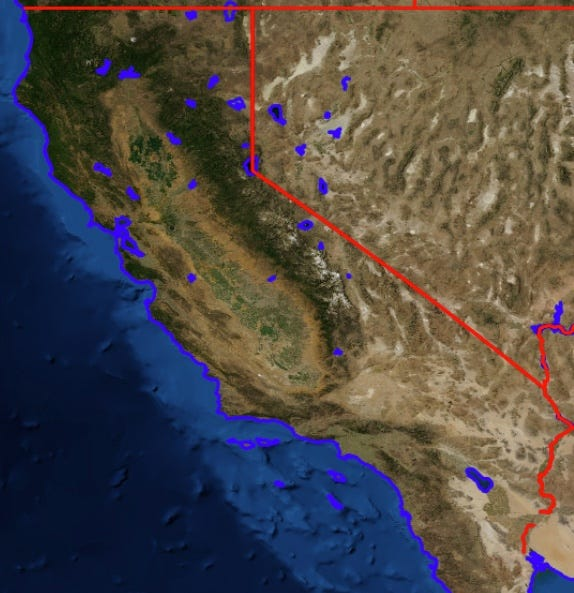The Image that is returned from above code

Now you could use this to plot a more specific area. For example, if you just wanted to plot city size, you could easily do that. Below is an example of this. Here is the link for the data for the below plot, in this case, I’m just plotting the location of the cities with the size being proportional to the density of the city.

import pandas as pd
import numpy as np

# Read in data on all cities

# Choose only cities in california
cities = cities.loc[cities.state_id == 'CA',:]

# Get all the data from the dataframe
lat, lon = cities['lat'], cities['lng']
population,density = cities['population'], cities['density']

# Scatter the points, using size and color but no label
plt.scatter(lon, lat, label=None,
c=np.log10(population),s= 10*np.log(density),
cmap='viridis', linewidth=0, alpha= .4)
plt.axis(aspect='equal')
plt.xlabel('longitude')
plt.ylabel('latitude')
plt.colorbar(label='log$_{10}$(population)')
plt.clim(3, 7)

# make a guide for the user
for density in [1, 50//3, 50]:
plt.scatter([], [], c='k', alpha=0.3, s=5*np.log(density),
label='10* log('+str(density) + ')')
plt.legend(scatterpoints=1, frameon=False, labelspacing=1, title='City Density')

plt.title('California Cities: Density and Population');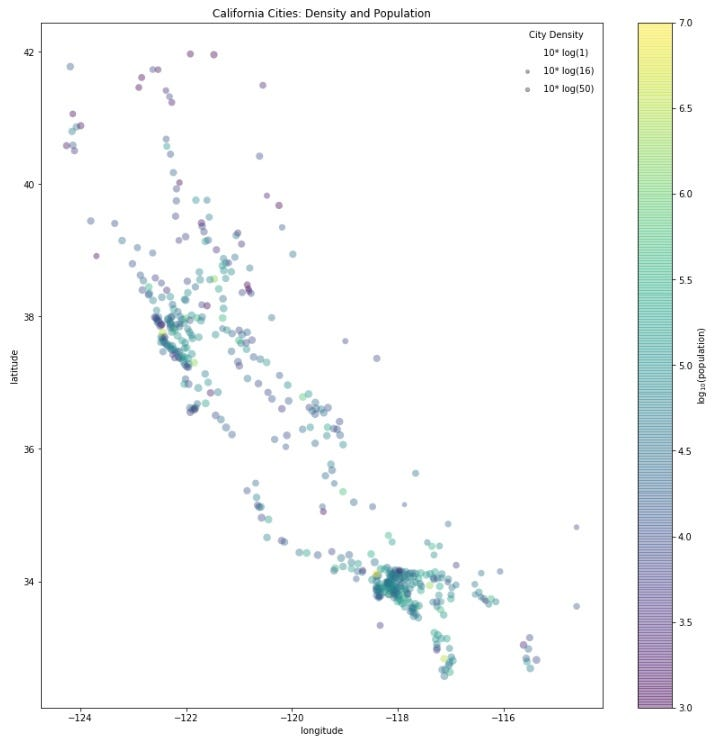Plotted the Cities in California, but now we need to add it to the basemap

Putting these two pieces together, we can see how easy it is to make a beautiful map that people can now gain a lot of information from.

# Nessecary Imports
import matplotlib.pyplot as plt
from mpl_toolkits.basemap import Basemap

# Make the figure
plt.figure(figsize=(14, 14))
# Initialize the basemap
m = Basemap(llcrnrlat = 30,
llcrnrlon = -126,
urcrnrlat = 45,
urcrnrlon = -114,
resolution='h')

# Get the area of interest imagery
m.arcgisimage(service='ESRI_Imagery_World_2D', xpixels = 2500, verbose= True,alpha= .6)

# Draw the coasts
m.drawcoastlines(color='blue', linewidth=1)

# Draw the states
m.drawstates(color='red',linewidth=3)
m.scatter(lon, lat, latlon=True,
c=np.log10(population), s=10*np.log(density),
cmap='Reds', alpha=0.5)
# 3. create colorbar and legend
plt.colorbar(label=r'$\log_{10}({\rm population})$')
plt.clim(3, 7)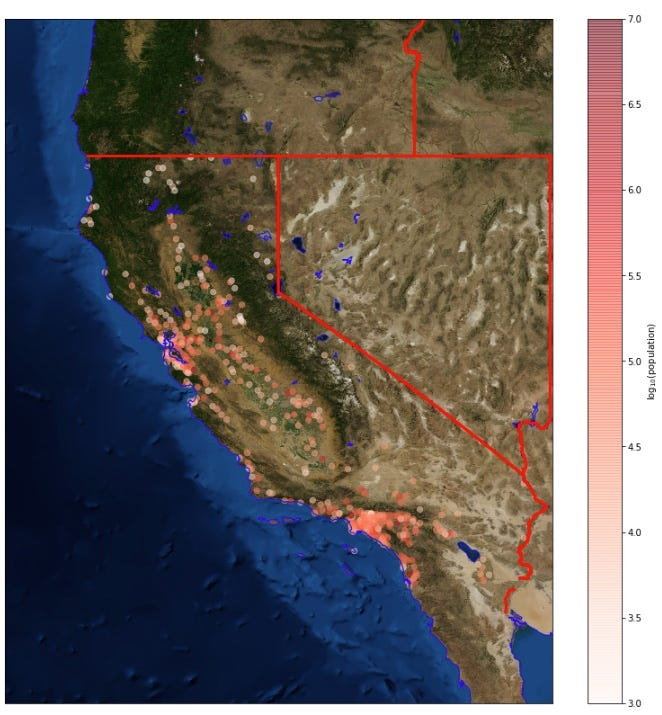Nice Image representing population

1. Now are going to take a look at live satellite data. This is a little bit more in depth, but I am going to just show you where to get the data, and how it looks after plotting it onto our map.The links to the live satellite data are below. There are 3 datasets from the infrared satellites. Anomalies in the last 24h, last 48hr, and last 7d.

This image was taken on July 31st, and all the code to achieve this plot is below.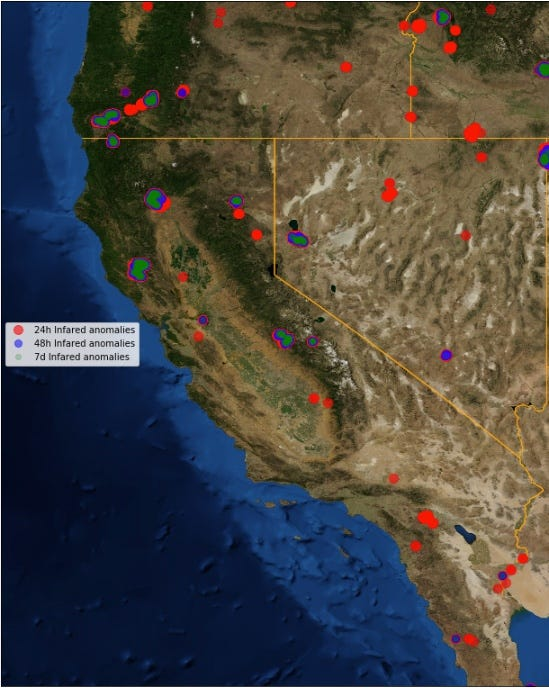Infrared anomalies

And you can zoom in closer to a specific fire to see more (Carr fire near Redding CA)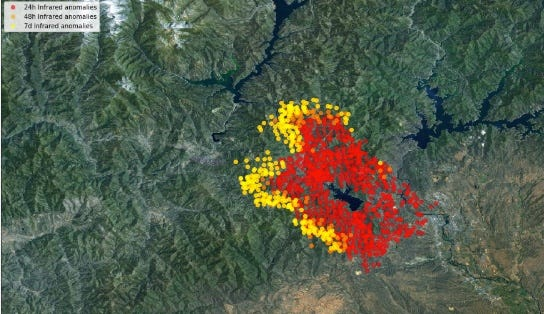And here is all the code!

def make_folder_name(localtime):

if localtime.tm_hour > 12:
string = str(localtime.tm_mon)+'_'+str(localtime.tm_mday)+'__'+ str(localtime.tm_hour-12)+'-'+str(localtime.tm_min)+'_pm'

else:
string = str(localtime.tm_mon)+'_'+str(localtime.tm_mday)+'_'+ str(localtime.tm_hour)+'-'+str(localtime.tm_min)+'_am'
return string
import zipfile
import requests
import time
#names of the datasets and their respective links
names = ['24hrModis1km','48hrModis1km','7dModis1km']
'https://firms.modaps.eosdis.nasa.gov/active_fire/c6/shapes/zips/MODIS_C6_USA_contiguous_and_Hawaii_48h.zip',
'https://firms.modaps.eosdis.nasa.gov/active_fire/c6/shapes/zips/MODIS_C6_USA_contiguous_and_Hawaii_7d.zip']
folder_names = []
localtime = time.localtime(time.time())
# Save the data into the right spot
# Go through each and  link

# open method to open a file on your system and write the contents
code.write(r.content)

# Unzip the data
folder_names.append(name)
zip_ref.extractall(name)
zip_ref.close()

import matplotlib.pyplot as plt
from mpl_toolkits.basemap import Basemap
import shapefile
from geopy.geocoders import Nominatim
geolocator = Nominatim()
plt.figure(figsize=(14, 14))
m = Basemap(llcrnrlat = 30,
llcrnrlon = -126,
urcrnrlat = 45,
urcrnrlon = -114,
resolution='h')
print('fetching image')
m.arcgisimage(service='ESRI_Imagery_World_2D', xpixels = 2500, verbose= True,alpha= .6)
# Draw the states
m.drawstates(color='orange',linewidth=1)
names2 = ['MODIS_C6_USA_contiguous_and_Hawaii_24h','MODIS_C6_USA_contiguous_and_Hawaii_48h','MODIS_C6_USA_contiguous_and_Hawaii_7d']
i = 1
for name,name1 in (zip(reversed(folder_names),reversed(names2))):
shpFilePath = name+'/'+name1
listx=[]
listy=[]
for sr in test.shapeRecords():
x,y = (sr.shape.points)
listx.append(x)
listy.append(y)
x,y = m(listx,listy)
if i == 1:
Color = 'r'
Label = '24h Infrared anomalies'
a = .6
m.plot(x, y, 'o',color = Color, markersize=10,alpha = a,label=Label)
if i == 2:
Color = 'b'
Label = '48h Infrared anomalies'
a = .5
m.plot(x, y, 'o',color = Color, markersize=8,alpha = a,label=Label)
if i == 3:
Color = 'g'
Label = '7d Infrared anomalies'
a = .2
m.plot(x, y, 'o',color = Color, markersize=6,alpha = a,label=Label)

i = i + 1
plt.legend()